# The Universe of Discourse

Tue, 13 Feb 2007

Cycle classes of permutations
I've always had trouble sleeping. In high school I would pass the time at night by doing math. Math is a good activity for insomniacs: It's quiet and doesn't require special equipment.

This also makes it a good way to pass the time on trains and in boring meetings. I've written before about the time-consuming math problems I use to pass time on trains.

Today's article is about another entertainment I've been using lately in meetings: count the number of permutations in each cycle class.

In case you have forgotten, here is a brief summary: a permutation is a mapping from a set to itself. A cycle of a permutation is a subset of the set for which the elements fall into a single orbit. For example, the permutation:

$$\pmatrix{1&2&3&4&5&6&7&8\cr 1&4&2&8&5&7&6&3\cr}$$

can be represented by the following diagram: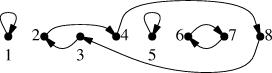And, since it contains four cycles (the closed loops), it is the product of the four cycles (1), (2 4 8 3), (5), and (6 7).

We can sort the permutations into cycle classes by saying that two permutations are in the same cycle class if the lengths of the cycles are all the same. This effectively files the numeric labels off the points in the diagrams. So, for example, the permutations of {1,2,3} fall into the three following cycle classes:

 Cycle lengths Permutations How many?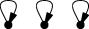1 1 1 () 1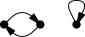2 1 (1 2)(1 3)(2 3) 3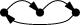3 (1 2 3)(1 3 2) 2

Here's the corresponding table for permutations of {1,2,3,4}:

 Cycle lengths Permutations How many?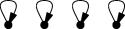1 1 1 1 () 1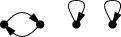2 1 1 (1 2)(1 3)(1 4) (2 3)(2 4)(1 4) 6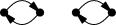2 2 (1 2)(3 4)(1 3)(2 4)(1 4)(2 3) 3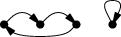3 1 (1 2 3)(1 2 4) (1 3 2)(1 3 4) (1 4 2)(1 4 3) (2 3 4)(2 4 3) 8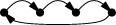4 (1 2 3 4) (1 2 4 3) (1 3 2 4) (1 3 4 2) (1 4 2 3) (1 4 3 2) 6

Counting up the number of permutations in each cycle class and coming up with a theorem about it was a good way to kill an hour or two of meeting time. It has a built-in check, which is that the total counts of all the cycle classes for permutations of N things had better add up to N!, or else you know you have made a mistake.

It is not too hard a problem, and would probably only take fifteen or twenty minutes outside of a meeting, but this is exactly what makes it a good problem for meetings, where you can give the problem only partial and intermittent attention. Now that I have a simple formula, the enumeration of cycle classes loses all its entertainment value. That's the way the cookie crumbles.

Here's the formula. Suppose we want to know how many permutations of {1,...,n} are in the cycle class C. C is a partition of the number n, which is to say it's a multiset of positive integers whose sum is n. If C contains p1 1's, p2 2's, and so forth, then the number of permutations in cycle class C is:

$$N(C) = {n! \over {\prod i^{p_i}{p_i}!}}$$

This can be proved by a fairly simple counting argument, plus a bit of algebraic tinkering. Note that if any of the pi is 0, we can disregard it, since it will contribute a factor of i0·0! = 1 in the denominator.

For example, how many permutations of {1,2,3,4,5} have one 3-cycle and one 2-cycle? The cycle class is therefore {3,2}, and all the pi are 0 except for p2 = p3 = 1. The formula then gives 5! in the numerator and factors 2 and 3 in the denominator, for a total of 120/6 = 20. And in fact this is right. (It's equal to !!2{5\choose3}!!: choose three of the five elements to form the 3-cycle, and then the other two go into the 2-cycle. Then there are two possible orders for the elements of the 3-cycle.)

How many permutations of {1,2,3,4,5} have one 2-cycle and three 1-cycles? Here we have p1 = 3, p2 = 1, and the other pi are 0. Then the formula gives 120 in the numerator and factors of 6 and 2 in the denominator, for a total of 10.

Here are the breakdowns of the number of partitions in each cycle class for various n:

 1 1 1 2 1 1 1 2 1 3 1 1 1 1 1 2 3 3 2 4 1 1 1 1 1 1 1 2 6 2 2 3 3 1 8 4 6 5 1 1 1 1 1 1 2 1 1 1 10 2 2 1 15 3 1 1 20 3 2 20 4 1 30 5 24 6 1 1 1 1 1 1 1 2 1 1 1 1 15 2 2 1 1 45 2 2 2 15 3 1 1 1 40 3 2 1 120 3 3 40 4 1 1 90 4 2 90 5 1 144 6 120
I find it a bit surprising that the most common cycle structure for permutations of 6 elements is to have one element map to itself and the others in one big 5-cycle. But on the other hand, there's a well-known theorem that the average permutation has exactly one fixed point, and so perhaps I shouldn't be surprised that the most likely cycle structure also has exactly one fixed point.

Incidentally, the thing about the average permutation having exactly one fixed point is quite easy to prove. Consider a permutation of N things. Each of the N things is left fixed by exactly (N-1)! of the permutations. So the total number of fixed points in all the permutations is N!, and we are done.

A similar but slightly more contorted analysis reveals that the average number of 2-cycles per permutation is 1/2, the average number of 3-cycles is 1/3, and so forth. Thus the average number of total cycles per permutation is !!\sum_{i=1}^n{1\over i} = H_n!!. For example, for n=4, examination of the table above shows that there is 1 permutation with 4 independent cycles (the identity permutation), 6 with 3 cycles, 11 with 2 cycles, and 6 with 1 cycle, for an average of (4+18+22+6)/24 = 50/24 = 1 + 1/2 + 1/3 + 1/4.

The 1, 6, 11, 6 are of course the Stirling numbers of the first kind; the identity !!\sum{n\brack i}i = n!H_n!! is presumably well-known.